Next: 2.1.2 Phononic Structure Up: 2.1 Graphene Previous: 2.1 Graphene   Contents

## 2.1.1 Electronic Structure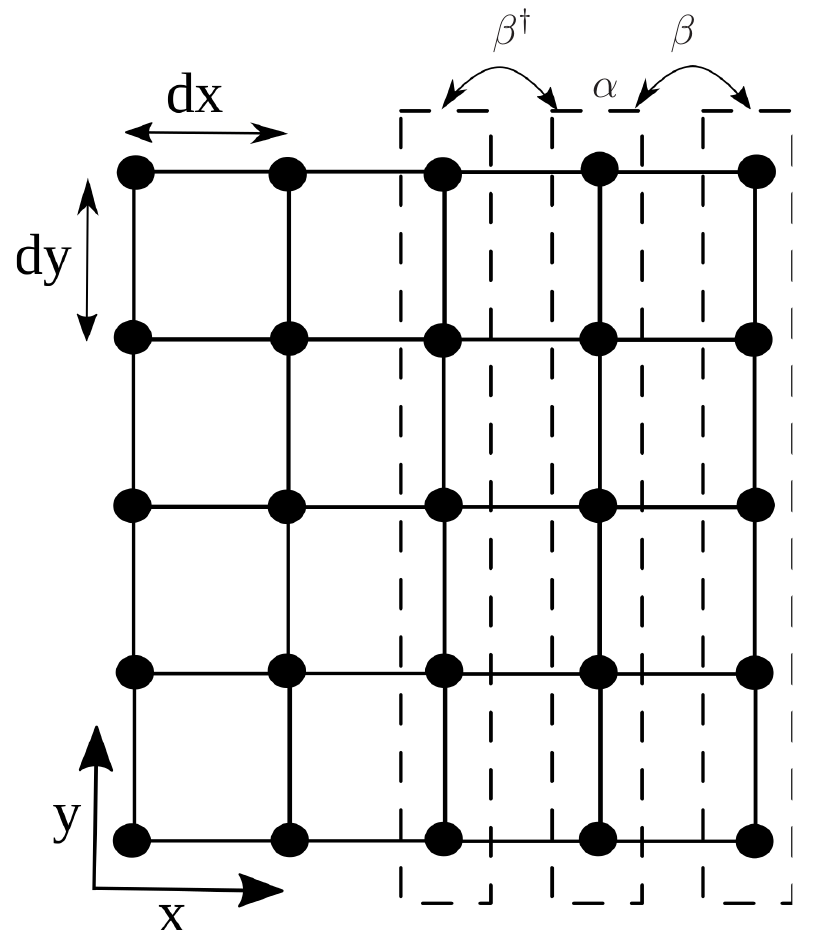The empirical tight-binding model is a standard, convenient, and accurate method for calculating the electronic structure of semiconductors. It is also referred to as Bloch or linear combination of atomic orbitals (LCAO) method . The Hamiltonian matrix for a simplified 2D system, using the TB method is described as follows: The 2D structure in Fig. 2.1 is composed of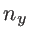chains of lattice points (i.e. atomic sites), each chain with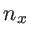sides. Assuming that each point is represented by one basis orbital, the Hamiltonian matrix will have the size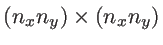, and is given by:(2.2)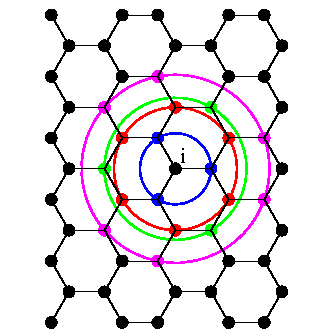where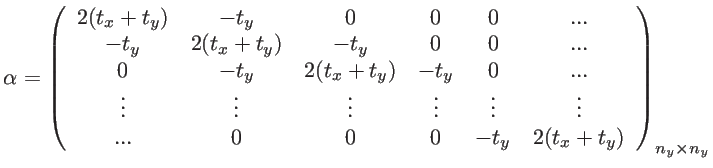(2.3)

and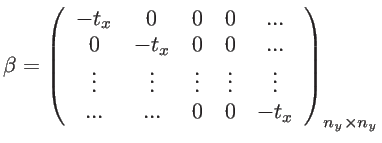(2.4)

where the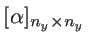submatrix describes the coupling within each chain, and the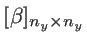submatrix describes the coupling between the adjacent chains. In general, the size of these components would be, where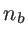is the number of basis orbitals in each unit cell. The eigenvalues of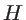are the corresponding energies for the electrons in the structure and can be adjusted by fitting the parameters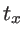and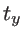. In the case of an open system, the boundary conditions are implemented by modifying the Hamiltonian to: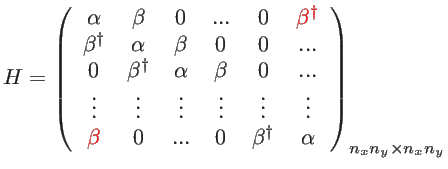(2.5)

The only change, here, is in the submatrices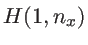and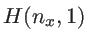, which now include the self energies of the left and right contacts. In the case of periodic boundary conditions, the bandstructure is calculated by considering the unit cell (index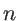) of the lattice, connected to the neighboring unit cells (index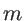) using the matrix elements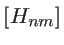. For example, as indicated in the structure of Fig. 2.1, once periodic boundary conditions are applied along the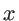-axis, then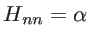and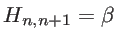for all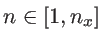. The bandstructure of the lattice is then obtained by calculating the eigenvalues of the Hamiltonian as: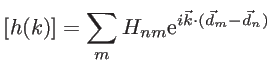(2.6)

for each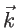-point in the Brillouin zone (BZ) . Here,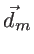is the vector corresponding to the position of neighboring unit celland the sum is over all neighboring unit cells.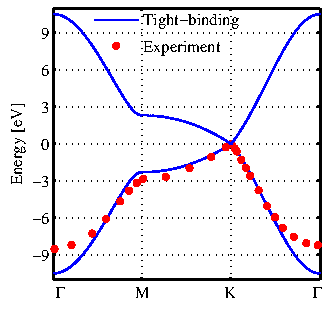In the case of graphene, a third nearest neighbor tight-binding model is used to describe its electronic structure. In this case, the particular atom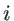and its nearest neighbor atoms are shown in Fig. 2.2. The hopping parameter between two nearest atoms separated by distance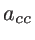is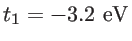. The tight-binding parameter of the third-nearest neighbor atoms located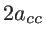away from each other is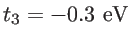. The hopping parameter for the second nearest-neighbor is assumed to be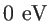. The bandstructure of graphene along the high-symmetry band line is shown in Fig. 2.3. The tight-binding results are in good agreement with experimental data taken from , in particular around the Fermi energy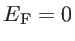which dominates the electrical properties. As shown in Ref.  this method can capture the details of the bandstructure of graphene-based nanostructures. The tight-binding model with calibrated parameters provides band-gap and subband-edge energies in excellent agreement with first-principles calculations .Next: 2.1.2 Phononic Structure Up: 2.1 Graphene Previous: 2.1 Graphene   Contents
H. Karamitaheri: Thermal and Thermoelectric Properties of Nanostructures